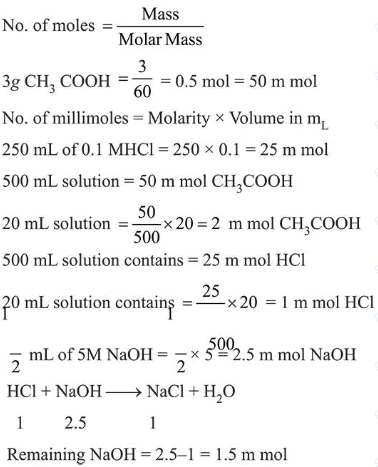# Solve the followingQuestion:

$3 \mathrm{~g}$ of acetic acid is added to $250 \mathrm{~mL}$ of $0.1 \mathrm{M} \mathrm{HCI}$ and the solution made up to $500 \mathrm{~mL}$. To $20 \mathrm{~mL}$ of this solution $\frac{1}{2} \mathrm{~mL}$ of $5 \mathrm{M} \mathrm{NaOH}$ is added. The $\mathrm{pH}$ of the solution is___________________.

[Given: pKa of acetic acid $=4.75$, molar mass of acetic acid $=60 \mathrm{~g} / \mathrm{mol}, \log 3=0.4771$ ]

Neglect any changes in volume.

Solution:

(5.22)